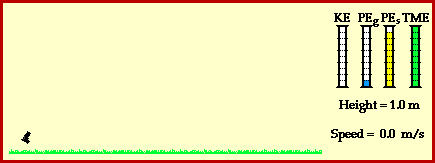## Energy Transformation for a Dart

Consider an ordinary dart projected from a toy dart gun and moving through the air. How could work and energy be utilized to analyze the motion of the dart? Would the total mechanical energy of the dart/gun system be altered when launched or while moving through the air? Or would the total mechanical energy of the dart/gun merely be conserved?

Of course the answers to these questions begin by determining whether or not there are any external forces doing work upon the dart/gun system. If external forces do work upon the dart/gun system, the total mechanical energy of the dart is not conserved; the initial amount of mechanical energy is not the same as the final amount of mechanical energy. On the other hand, if external forces do not do work upon the dart/gun system, then the total mechanical energy is conserved; that is, mechanical energy is merely transformed from one form to another (say from potential to kinetic and/or vice versa) while the total amount of the two forms remains unchanged.

In this case of the dart being launched from the spring gun, the only forces doing work upon the dart are internal forces. Initially, the dart is being acted upon by a spring force in order to be projected from the dart gun. The coils of the springs are initially compressed and upon pulling the trigger, the springs return to their equilibrium position while pushing the dart out of the dart gun. The dart then becomes a projectile (assuming there is negligible air resistance); the only force doing work upon the dart during its flight through the air is gravity. Since both the spring force and the force of gravity are internal forces, the total mechanical energy of the dart is conserved. The animation below depicts the motion of the dart. The animation is accompanied by work-energy bar charts which further illustrate the transformation of energy from one form to another and the conservation of the total amount of mechanical energy.The animation above shows that the energy of the dart/gun system is initially present in the form of the elastic potential energy (PEs) and gravitational potential energy (PEg). The springs of the dart gun are compressed which accounts for the elastic potential energy. Furthermore, the dart is initially elevated at a height of 1-meter above the ground which accounts for the gravitational potential energy. The presence of these two initial forms of energy are shown by the PEg and PEs bars of the bar chart. Once projected, the dart no longer has elastic potential energy since the springs of the dart are no longer compressed. However, the dart does have a large amount of kinetic energy (energy of motion) since it is now moving at a high speed as it leaves the dart. The dart also has gravitational potential energy since it is still elevated to some height above the ground. As the dart ascends towards its peak, it is continuously slowing down under the influence of the downward force of gravity. During this ascent, there is a transformation of the mechanical energy from the form of kinetic energy (energy of motion) to gravitational potential energy (the stored energy of vertical position). At the peak, there is only a small amount of kinetic energy (the dart still has a horizontal motion) and a large amount of gravitational potential energy (the dart is at its highest vertical position). Finally, as the dart descends to the ground, the force of gravity speeds it up. As it falls, there is an increase in kinetic energy (due to the gain in speed) and a decrease in gravitational potential energy (due to a loss in vertical position).

The above animation provides a simple demonstration of how mechanical energy merely changes form when the only forces acting upon an object are internal forces. While the form of mechanical energy changes, the total amount of mechanical energy (TME) is conserved.

For more information on physical descriptions of motion, visit The Physics Classroom Tutorial. Detailed information is available there on the following topics:

Potential Energy

Kinetic Energy

Mechanical Energy

Internal vs. External Forces

Work

Work-Energy Theorem

Work-Energy Bar Charts